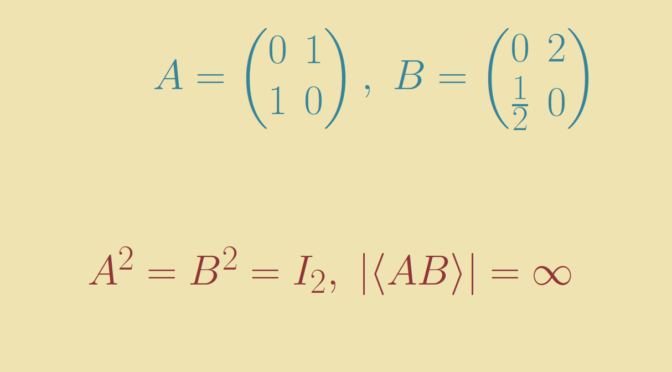# Subset of elements of finite order of a group

Consider a group $$G$$ and have a look at the question: is the subset $$S$$ of elements of finite order a subgroup of $$G$$?

The answer is positive when any two elements of $$S$$ commute. For the proof, consider $$x,y \in S$$ of order $$m,n$$ respectively. Then $\left(xy\right)^{mn} = x^{mn} y^{mn} = (x^m)^n (y^n)^m = e$ where $$e$$ is the identity element. Hence $$xy$$ is of finite order (less or equal to $$mn$$) and belong to $$S$$.

### Example of a non abelian group

In that cas, $$S$$ might not be subgroup of $$G$$. Let’s take for $$G$$ the general linear group over $$\mathbb Q$$ (the set of rational numbers) of $$2 \times 2$$ invertible matrices named $$\text{GL}_2(\mathbb Q)$$. The matrices $A = \begin{pmatrix}0&1\\1&0\end{pmatrix},\ B=\begin{pmatrix}0 & 2\\\frac{1}{2}& 0\end{pmatrix}$ are of order $$2$$. They don’t commute as $AB = \begin{pmatrix}\frac{1}{2}&0\\0&2\end{pmatrix} \neq \begin{pmatrix}2&0\\0&\frac{1}{2}\end{pmatrix}=BA.$ Finally, $$AB$$ is of infinite order and therefore doesn’t belong to $$S$$ proving that $$S$$ is not a subgroup of $$G$$.